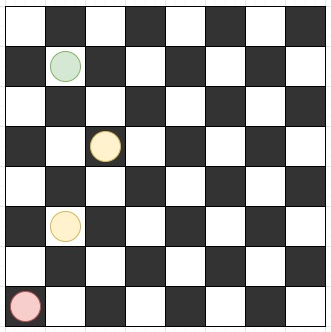# Program to find minimum steps to reach target position by a chess knight in Python

Suppose we have two values r and c. If a chess knight is placed at the coordinate (0, 0) at the beginning in an infinitely large chess board, we have to find minimum number of moves it would take to reach the location (r, c). The knight will follow same moving style as chess. It moves two squares away horizontally and one square vertically, or two squares vertically and one square horizontally.

So, if the input is like r = 6, c = 1, then the output will be 3, the red is initial position, green is final and yellows are intermediate steps.To solve this, we will follow these steps −

• if r < c, then
• swap r and c
• if (r, c) is same as (1, 0), then
• return 3
• if (r, c) is same as (2, 2), then
• return 4
• delta := r - c
• if c > delta, then
• return delta - 2 *(quotient of (delta - c) / 3)
• otherwise,
• return delta - 2 *(quotient of (delta - c) / 4)

Let us see the following implementation to get better understanding −

## Example

Live Demo

class Solution:
def solve(self, r, c):
if r < c:
r, c = c, r
if (r, c) == (1, 0):
return 3
if (r, c) == (2, 2):
return 4
delta = r - c
if c > delta:
return delta - 2 * ((delta - c) // 3)
else:
return delta - 2 * ((delta - c) // 4)
ob = Solution()
r = 6
c = 1
print(ob.solve(r, c))

## Input

6, 1

## Output

3